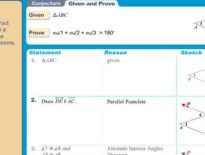# 6th-8th Grade Math Activity: Algebra Tiles Explained

Algebra tiles can help students visualize and understand the process of solving equations. This interactive activity breaks down each step of the process for solving algebra problems.

This course can be found in our Fundamentals of Geometry and Algebra Course

Course Details:

Students enhance computational and problem-solving skills while learning topics in algebra, geometry, probability, and statistics. They solve expressions and equations in the context of perimeter, area, and volume problems while further developing computational skills with fractions and decimals. The study of plane and solid figures includes construction and transformations of figures. Also in the context of problem solving, students add, subtract, multiply, and divide positive and negative integers and solve problems involving ratios, proportions, and percents, including simple and compound interest, rates, discount, tax, and tip problems. They learn multiple representations for communicating information, such as graphs on the coordinate plane, statistical data and displays, as well as the results of probability and sampling experiments. They investigate patterns involving addition, multiplication, and exponents, and apply number theory and computation to mathematical puzzles.

Request a free catalog of K-12 Courses

Request the Scope & Sequence for a course

## 6th-8th Grade Math Activity: Algebra Tiles Explain…